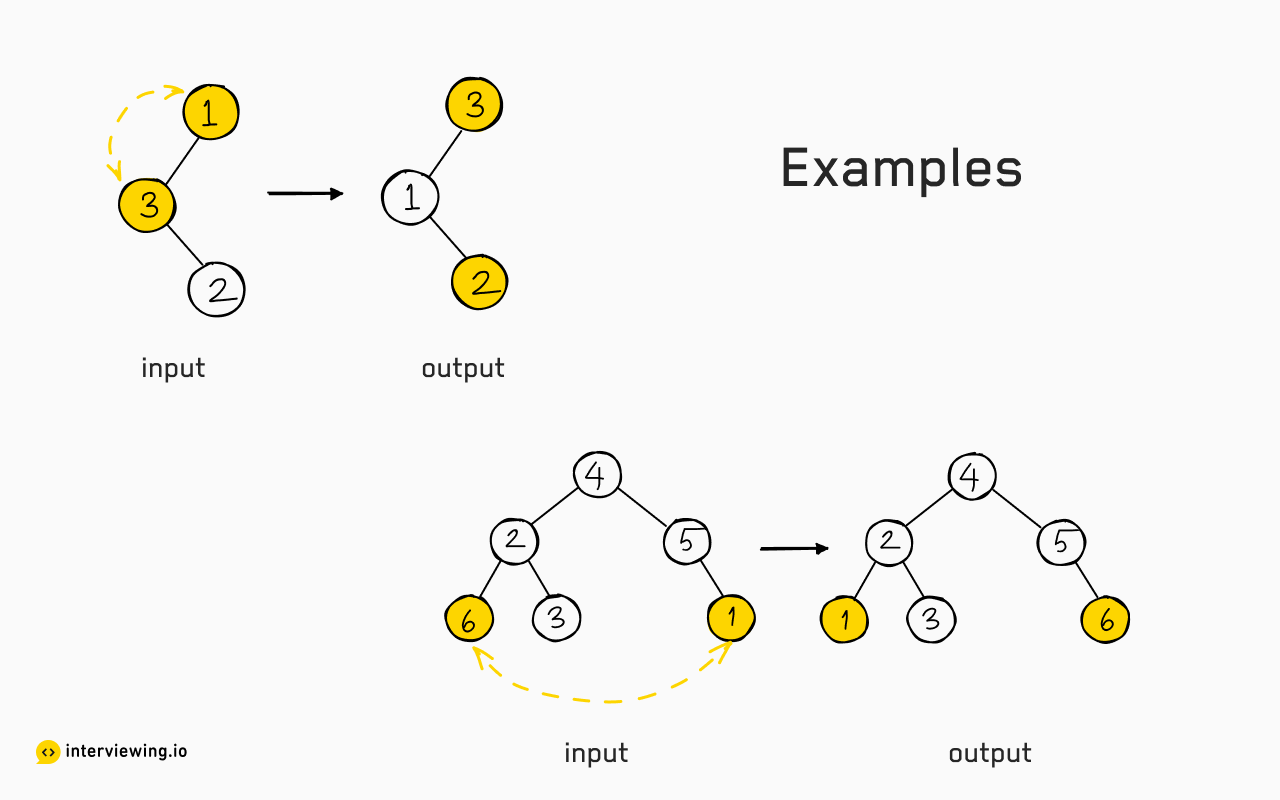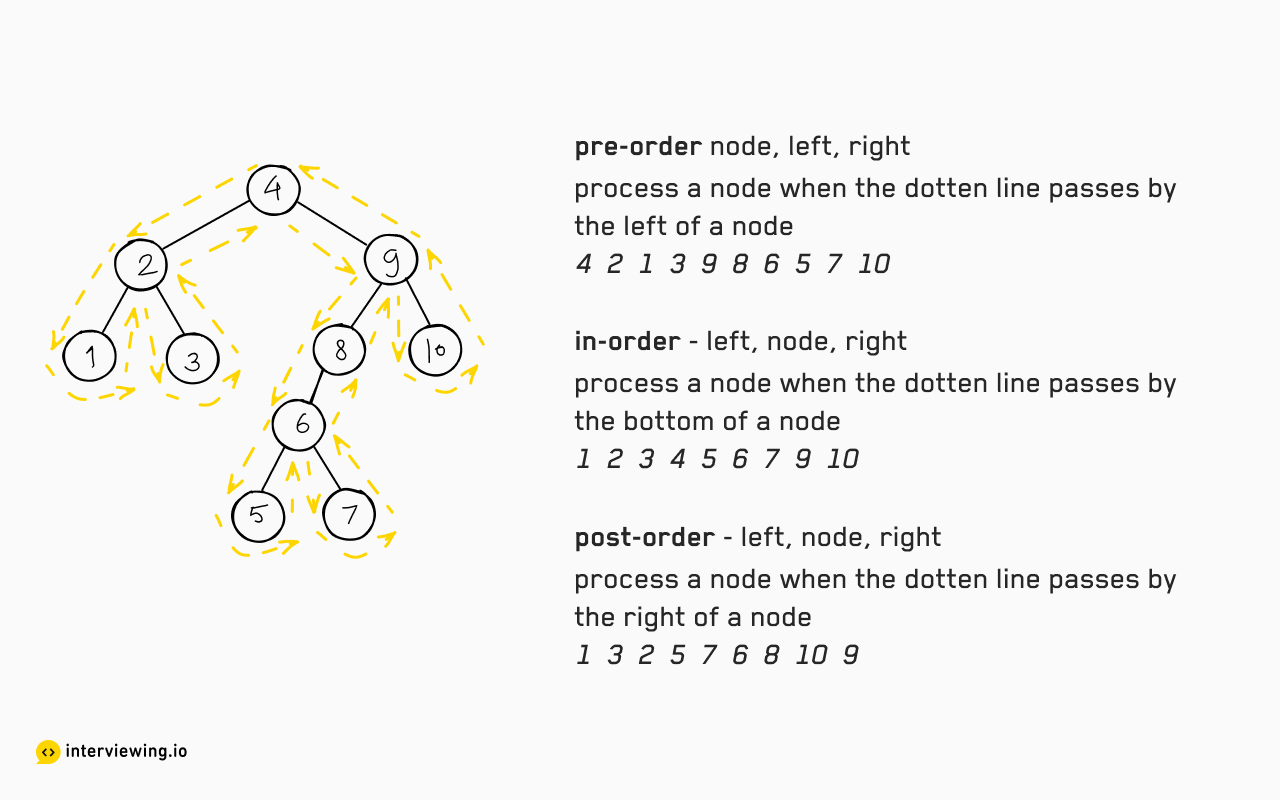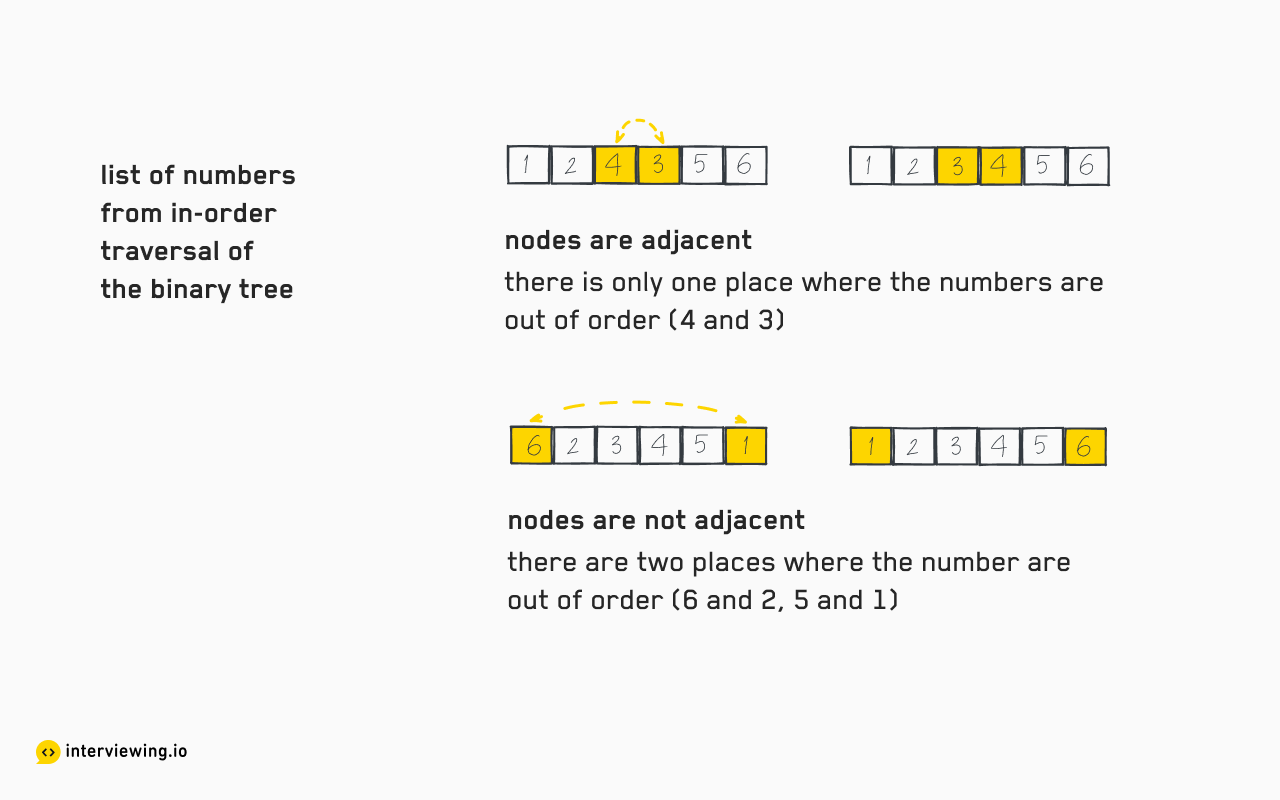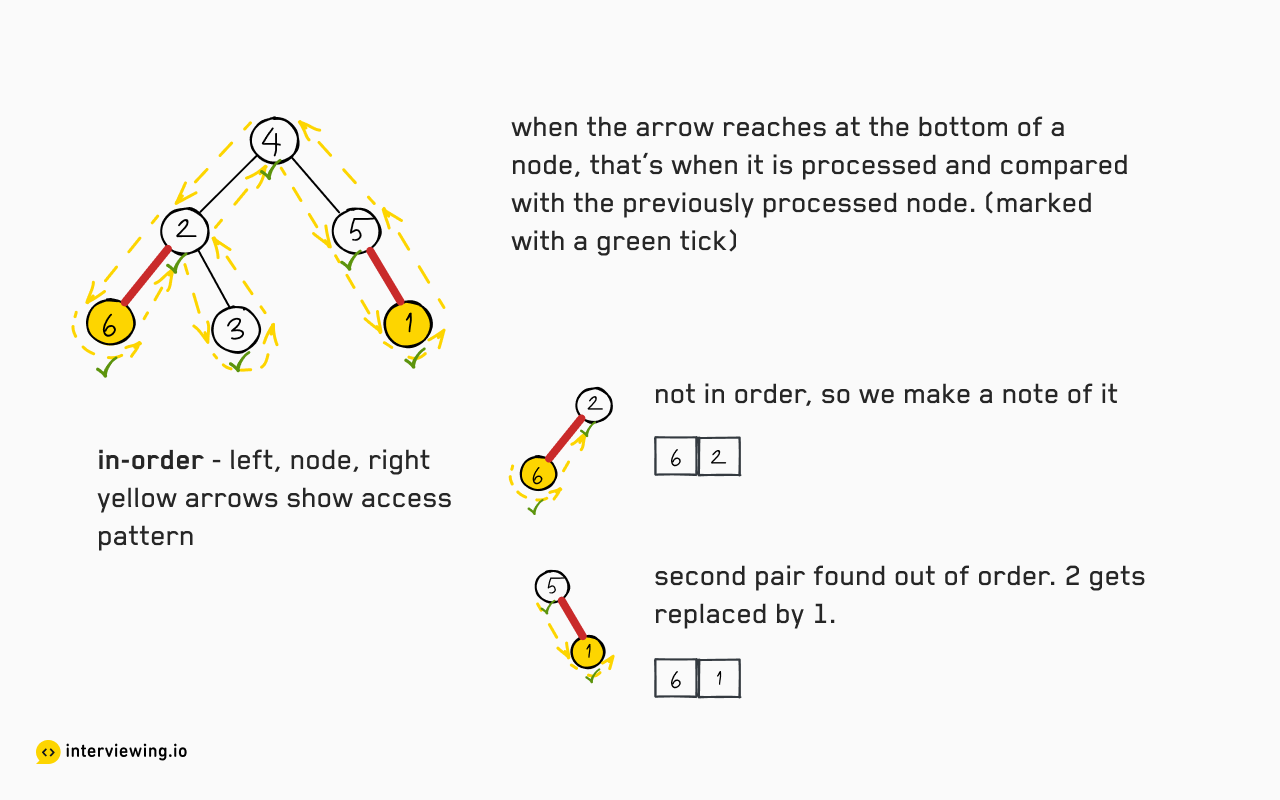MEDIUM
DATA STRUCTURES AND ALGORITHMS

# How to Solve Recover Binary Search Tree

Written ByKenny PolyakJai Pandya

## Recover Binary Search Tree Introduction

The Recover Binary Search Tree problem asks us to restore a binary search tree to its original form after two of its nodes have been swapped. This problem requires a strong understanding of the structure and properties of a binary search tree, namely that all nodes to the left of the root are smaller than the root and all nodes to the right are larger.

## Recover Binary Search Tree Problem

### Example Inputs and Outputs#### Example 1

Input:

``````    1
/
3
\
2
``````

Output:

``````    3
/
1
\
2
``````

#### Example 2

Input:

``````     4
/ \
2   5
/ \   \
6   3   1
``````

Output:

``````     4
/ \
2   5
/ \   \
1   3   6
``````

Constraints

• The number of nodes in this tree is in the range [2, 1000]
• -5000 <= Node.val <= 5000

## Recover Binary Search Tree Solutions

#### Overview and Intuition

In this question, we are provided with the root of a binary search tree, well, an almost binary search tree. Someone accidentally swapped two of its nodes, turning the BST into a regular binary tree. We are on a quest to find those two nodes. Once we find them, it shouldn't be difficult to swap them again to restore the original BST.

Let's first understand the characteristics of this special kind of tree called a "binary search tree" or BST. The "special" part comes from the order in which it stores its nodes. As with a binary tree, each node of a BST can have at most two children, one left and one right. But each node maintains a special relationship with its children and parent.

``````class TreeNode:
def __init__(self, data):
self.data = data
self.left = None
self.right = None
``````

In a BST, the value of any node is greater than the value of all nodes in its left subtree. In contrast, the value of any node is smaller than the value of all nodes in its right subtree. All nodes are related in this way to their respective left and right subtrees.

Now that we have some clarity about BST, let's return to our original problem. It says that two of the nodes of the given BST have been swapped. Let's look at what a BST might look like if that were the case.

Here we can see that the original tree satisfies all the properties of a BST. As soon as the nodes with values `1` and `6` are swapped, they no longer satisfy these properties. `6` is larger than `2` and `4`, but it is located in the left subtree of the two nodes after the swap. Similarly, `1` is smaller than `5` and `4`, but it is in the right subtree of `5` and `4`.

We can take advantage of another interesting property of a binary search tree, which relates to its in-order 'depth first search' traversal. In in-order traversal, the left subtree is processed first, then the current node, and then the right subtree. We continue this recursively for each node. We already know that in a BST, the left child is always smaller, while the right child is always larger than the current node. The in-order traversal thus ensures that the nodes with smaller values are processed before those with higher values, i.e., in ascending sort order.For the given example - in-order traversal of the original configuration would produce the following array - `[1, 2, 3, 4, 5, 6]`. After swapping the two nodes, it changes to - `[6, 2, 3, 4, 5, 1]`

Starting with this basic idea, we will examine three approaches to this problem.

### Approach 1: Export to an Array - Two Pass Solution

#### Intuition

In the previous section, we learned about the idea of in-order traversal. We take it further and implement it in this approach. We traverse the given tree in an in-order fashion. When we process a node, we push its reference into a list. If this were a perfect BST, the list or array would be perfectly sorted, and all nodes would be in ascending order of their values. In our case, however, there are two defectors.

The problem has now changed slightly. Given a list of almost sorted nodes, we need to find the two nodes that are not in the correct order. Eventually, we will interchange their values.

There are two possibilities -

1. When the defectors are next to each other in the resultant array.

Example: `[1, 2, 4, 3, 5, 6]`

We can see that `4` and `3`, which are right next to each other, have swapped positions. The ascending order is broken only at one place, namely at the pair `(4, 3)`, which are also the two nodes we are trying to find.

2. When the defectors are at least one element apart in the output list.

Example: `[6, 2, 3, 4, 5, 1]`

In this case, the perfect sort is broken for two pairs of values - `(6, 2)` and `(5, 1)`. It turns out that `5` and `1` are the errant nodes.#### Algorithm

1. We traverse the given tree in the in-order sequence, and push the processed nodes into a new list `almostSorted`. In the solution here, we use the recursive DFS algorithm to simplify the implementation. We could also use the iterative version.
2. If there are `N` elements in the list, iterate the index `i` through `almostSorted` from `0` till `N - 2`.
3. For each pair `almostSorted[i]` and `almostSorted[i + 1]`, if they are not in ascending order, we note them in a variable `swapped`. If `swapped` already contains two nodes, it means that we have already encountered a pair out of order. This means that `almostSorted[i + 1]` must be the second defector.
4. Now that we have identified the two swapped elements, we just need to swap their values again.
5. Return the `root` of the binary tree, which has now become a perfect BST.

#### Code and Implementation

``````def recover_bst(self, root: Optional[TreeNode]) -> None:
almost_sorted = []
swapped = None

# traverse the given tree in in-order fashion
# and populate `almostSorted` array
def in_order(node: Optional[TreeNode]):
if node is None:
return

# Notice the order here
# 1. left child 2. current node 3. right child
in_order(node.left)
almost_sorted.append(node)
in_order(node.right)

in_order(root)

# `almost_sorted` is a sorted array except for the two
# nodes which have been swapped with each other. Find those
# nodes
for i in range(len(almost_sorted) - 1):
if almost_sorted[i].val > almost_sorted[i + 1].val:
if swapped is None:
swapped = [almost_sorted[i], almost_sorted[i + 1]]
else:
swapped = almost_sorted[i + 1]

# Exchange the value of the swapped nodes
swapped.val, swapped.val = (swapped.val, swapped.val)
return root
``````

#### Time/Space Complexity

• Time Complexity - `O(n)`, where `n` is the number of nodes in the tree. During in-order traversal, we iterate through every node in the tree to build the almost sorted list. Then once again, we iterate through all the elements. So this solution takes `O(n)` time. In other words, the time taken grows linearly with the size of the tree.
• Space Complexity - `O(n)`, where `n` is the number of nodes in the tree. We use an auxiliary data structure, a list, to store all processed nodes. It requires `O(n)` of memory. Recursion also requires space on the implicit stack, which can be as large as the height of the recursion tree - `O(n)` in the worst case. So this solution requires `O(n)` space on memory.

### Approach 2: In-order Recursive Traversal - Single Pass

#### Intuition

A tiny optimization over the naive approach can save us a pass through all nodes. In the previous solution, we create a list of nodes resulting from in-order traversal. But do we really need it?

The only purpose of the list is to give us easy access to the pairs of nodes `almostSorted[i]` and `almostSorted[i + 1]]` in order. However, we could also access them during the traversal. When we traverse the tree in in-order fashion, we can treat the current node as `almostSorted[i + 1]`, and its predecessor (the last node processed) represents `almostSorted[i]`. It turns out that it makes no sense to create an auxiliary list.

In a valid BST, the in-order predecessor should contain a smaller value than the current node. If we encounter a node that does not follow this rule, we note the predecessor and the current node. There can be, at most, two such nodes.#### Algorithm

Traverse the tree in order. We use a recursive DFS algorithm here. Each node compares its value to its in-order predecessor. The node processed last is the in-order predecessor of a node. We store it in a global variable `lastProcessed`.

Let's make a recursive function `inOrder` and pass `root` as the current `node`.

1. Termination condition / base case - when the current `node` is `null`, the function should simply return.
2. Recursively call `inOrder` with the left child as the current `node`.
3. If `lastProcessed` is empty, it indicates that the current node is the leftmost child of the original tree, so it has no predecessor. As a result, no comparisons as well.
4. If `lastProcessed` is not empty - compare its value to the current node. If `lastProcessed.value > node.value`, then do the following: We store the pair `lastProcessed` and `node` in a two cell array `swapped`. If `swapped` already contains an element, it means the current pair is the second pair in our search. So we store `node` at `swapped`.
5. Update `lastProcessed` to the current node.
6. Make a recursive call to `inOrder` with the right child as the current `node`.
7. At the end, we swap the values of the nodes contained in `swapped`.
8. Return the `root` of the tree.

#### Code and Implementation

``````def recover_bst(self, root: Optional[TreeNode]) -> None:
swapped = last_processed = None

# traverse the given tree recursively in
# in-order fashion. left => current => right
# and find the pair with swapped nodes
def find_swapped_pair(current: Optional[TreeNode]):
nonlocal swapped, last_processed
if current is None:
return
find_swapped_pair(current.left)

# compare the current nodes with its predecessor
# there are two such nodes, so we find the condition
# to be true at most two times
if last_processed is not None:
if last_processed.val > current.val:
if swapped is None:
# encounter an out of order node for the first time
swapped = [last_processed, current]
else:
# encounter out of order node second time
swapped = current

last_processed = current
find_swapped_pair(current.right)

find_swapped_pair(root)

# exchange the values of both the nodes
swapped.val, swapped.val = swapped.val, swapped.val
return root
``````

#### Time/Space Complexity

• Time Complexity - `O(n)`, where `n` is the number of nodes in the tree. We traverse through all nodes in the tree. So, time taken in processing the given tree increases linearly with the tree size. The time complexity is `O(n)`.

• Space Complexity - `O(n)`, where `n` is the number of nodes in the tree. We do not create a list of processed nodes, but the recursive implementation requires memory on the implicit recursion stack. The size of the recursion stack can grow to the height of the tree. When the tree is balanced, the height is `log n`. In the worst case, when each node has only one child, `O(n)` memory is required on the recursion stack. Thus, the space complexity is `O(n)`.

### Approach 3: In-order Iterative Traversal - Single Pass

#### Intuition

We have already seen a recursive implementation of the solution. By using an explicit stack in place of an implicit recursion stack, we can convert the solution to use iteration instead of recursion.

We try to mimic what happens in the recursive implementation. During in-order traversal we process the left children before the current node. So we push the current node into a stack and move to the left child. In other words, we update the current pointer to point to the left child. We repeat this process until we reach a node without a left child. At this point because the left child is empty, we can process the current element.

After processing the current element, the order needs to move to the right side. So we update the current pointer to point to the right side node. Now, the current node cant be processed before its left children. So we push it into the stack and update the current pointer to point to its left child. We should see a pattern repeating here, which can be easily implemented using a loop.

In order to compare an element to its predecessor, we also keep track of the last processed node. The comparison happens right before the current pointer moves to the right side of a node.

#### Algorithm

Traverse the tree in in-order fashion. We use iteration here. Each node compares its value to its in-order predecessor. The node processed last is the in-order predecessor of a node. We store it in a global variable, `lastProcessed`.

Initialize an empty stack `stack`, and a pointer `current` pointing at the root node.

Initialize `lastProcessed` and `swapped` to contain `null` values.

Do the following until the stack is not empty or `current` points at a valid node:

1. While `current` points at a valid node a. Push the node pointed by `current` into the stack b. Update `current` to point to its left child

2. `current` should be pointing at `null` at this moment. So we update `current` by popping the last stored node from the stack.

3. If we have processed a node earlier, compare the current node's value with the last processed node. If we find the last processed node to have a value greater than the current node, we have found a node out of order. We put both `lastProcessed` and `current` into the array `swapped`.

If `swapped` already contains a node, it means the current node must be the second of the swapped pair. So we update `swapped` with the current node and end the traversal here. At the end, we update `lastProcessed` to point to the current node.

After traversal, we exchange the values of the nodes contained in `swapped` and return the `root` of the tree.

#### Code and Implementation

``````def recover_bst(self, root: Optional[TreeNode]) -> None:
stack = []
current = root
last_processed = swapped = None

# process until the stack contains something
# or the current pointer points to a node
while stack or current:

# reach as far left as possible and put all
# the nodes encountered into the stack
while current:
stack.append(current)
current = current.left

# current is None, repopulate it from the
# stack's top
current = stack.pop()

# critical step - compare the current node with the
# last processed node. If the node doesn't follow the
# expected order, note the pair of nodes
if last_processed and last_processed.val > current.val:
if swapped:
swapped = current
break
else:
swapped = [last_processed, current]

# update last_processed node
last_processed = current

# move to the right side
current = current.right

# swap the values of the two nodes
swapped.val, swapped.val = swapped.val, swapped.val
return root
``````

#### Time/Space Complexity

• Time Complexity - `O(n)`, where `n` is the number of nodes in the tree. We traverse through all nodes in the tree. So, the time taken to process the given tree increases linearly with the size of the tree. The time complexity is `O(n)`.
• Space Complexity - `O(n)`, where `n` is the number of nodes in the tree. The size of the stack used can grow to the height of the binary tree. When the tree is balanced, its height is `log n`. In the worst case, when each node has only one child, the tree height becomes `n`. Thus, `O(n)` memory is needed to accommodate the stack. Thus, the space complexity is `O(n)`.

#### Bonus - Morris Traversal

This approach may not be very relevant from the perspective of an interview. But it could be an interesting exercise if you want to try it out. We will not go into the details of this approach but will give you a basic idea here.

In the recursive and iterative versions described above, we consume `O(n)` of space on the implicit or explicit stack.

We use a stack to temporarily store the root nodes of different subtrees and the order in which they are processed. We put a node in the stack when we go down to the left child and remove it from the stack when we go back up. Could there be a way to skip the stack and still be able to go back up once we have examined the children of a node? This is precisely what we can do with Morris traversal.

As we traverse a binary tree in order, the predecessor makes a temporary connection to the next node. Once we have explored all the nodes of the left subtree, the connection between the predecessor and its successor helps us move back up (or down) the tree. After that, the predecessor is returned to its original shape.

This particular type of tree, where the predecessor nodes maintain a connection to their successor nodes, is also called a "threaded binary tree".

## Practice the Recover Binary Search Tree Problem With Our AI Interviewer

Start AI Interview